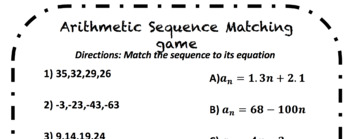# Arithmetic Sequence Matching worksheetSubject
Resource Type
File Type

PDF

(88 KB)
Product Rating
Standards
• Product Description
• StandardsNEW

This product can be given to each students or laminated for multiple uses.

Students can match the sequences to the explicit arithmetic sequence equation.

• 12 sequences
• 12 explicit equations
Construct linear and exponential functions, including arithmetic and geometric sequences, given a graph, a description of a relationship, or two input-output pairs (include reading these from a table).
Recognize that sequences are functions, sometimes defined recursively, whose domain is a subset of the integers. For example, the Fibonacci sequence is defined recursively by 𝘧(0) = 𝘧(1) = 1, 𝘧(𝘯+1) = 𝘧(𝘯) + 𝘧(𝘯-1) for 𝘯 greater than or equal to 1.
Total Pages
N/A
N/A
Teaching Duration
N/A
Report this Resource to TpT
Reported resources will be reviewed by our team. Report this resource to let us know if this resource violates TpT’s content guidelines.